Next: Dielectrics Up: Capacitance Previous: Breakdown

## Capacitance

As we have seen, the amount of charge which can be stored on a conductor is limited by the electric field-strength just above its surface, which is not allowed to exceed a certain critical value,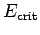. Unfortunately, the field-strength varies from point to point across the surface (unless the surface possesses a constant radius of curvature). It is, therefore, generally convenient to parameterize the maximum field-strength above the surface of a conductor in terms of the voltage differencebetween the conductor and either infinity or another conductor. The point is that, unlike the electric field-strength, is a constant over the surface, and can, therefore, be specified unambiguously.

How do we tell the difference between a good and a bad charge storage device? Well, a good charge storage device must be capable of storing a large amount of charge without causing breakdown. Likewise, a bad charge storage device is only capable of storing a small amount of charge before breakdown occurs. Thus, if we place a charge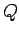in a good storage device then the electric fields generated just above the surface of the device should be comparatively weak. In other words, the voltageshould be relatively small. A convenient measure of the ability of a device to store electric charge is its capacitance,, which is defined as the ratio ofover: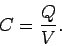(105)

Obviously, a good charge storage device possesses a high capacitance. Note that the capacitance of a given charge storage device is a constant which depends on the dimensions of the device, but is independent of eitheror. This follows from the linear nature of the laws of electrostatics: i.e., if we double the charge on the device, then we double the electric fields generated around the device, and so we double the voltage difference between the device and (say) infinity. In other words,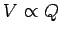. The units of capacitance are called farads (F), and are equivalent to coulombs per volt: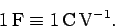(106)

A farad is actually a pretty unwieldy unit. In fact, most of the capacitors found in electronic circuits have capacitances in the micro-farad range.

Probably the simplest type of capacitor is the so-called parallel plate capacitor, which consists of two parallel conducting plates, one carrying a chargeand the other a charge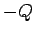, separated by a distance. Letbe the area of the two plates. It follows that the charge densities on the plates are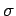and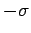, respectively, where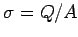. Now, we have already seen (in Sect. 4.5) that the electric field generated between two oppositely charged parallel plates is uniform, and of magnitude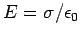. The field is directed perpendicular to the plates, and runs from the positively to the negatively charged plate. Note that this result is only valid if the spacing between the plates is much less than their typical dimensions. According to Eq. (73), the potential differencebetween the plates is given by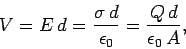(107)

where the positively charged plate is at the higher potential. It follows from Eq. (105) that the capacitance of a parallel plate capacitor takes the form(108)

Note that the capacitance is proportional to the area of the plates, and inversely proportional to their perpendicular spacing. It follows that a good parallel plate capacitor possesses closely spaced plates of large surface area.Next: Dielectrics Up: Capacitance Previous: Breakdown
Richard Fitzpatrick 2007-07-14